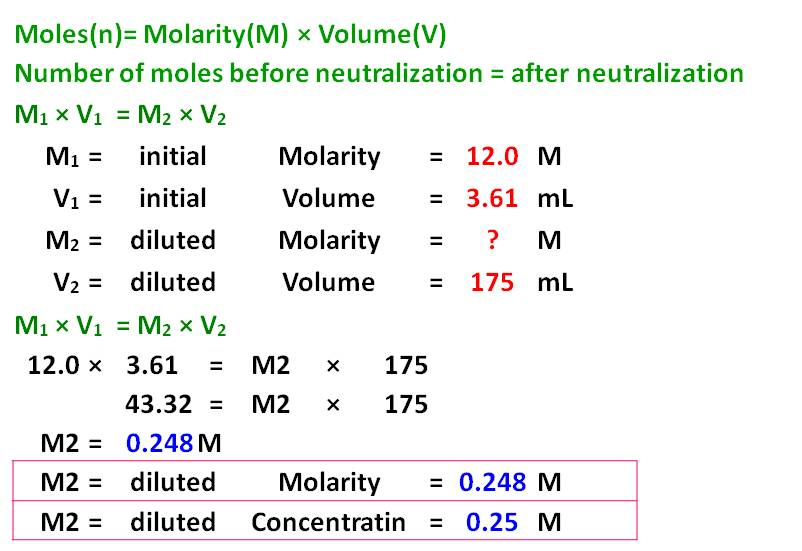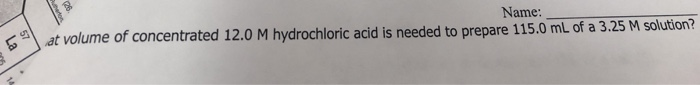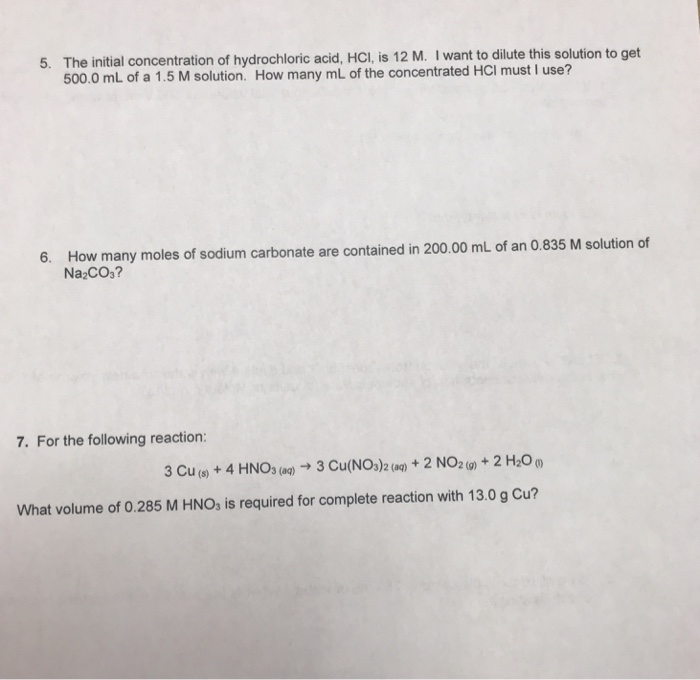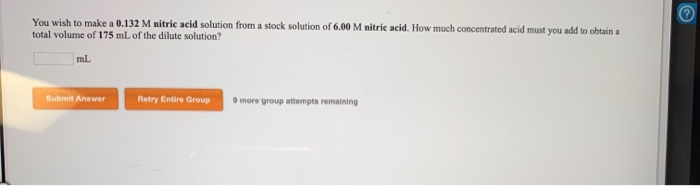Question

# In the laboratory, you dilute 3.61 mL of a concentrated 12.0 M hydrochloric acid solution to...

In the laboratory, you dilute 3.61 mL of a concentrated 12.0 M hydrochloric acid solution to a total volume of 175 mL. What is the concentration of the dilute solution?#### Earn Coins

Coins can be redeemed for fabulous gifts.

Similar Homework Help Questions
• ### You wish to make a 0.445 M hydrochloric acid solution from a stock solution of 12.0...

You wish to make a 0.445 M hydrochloric acid solution from a stock solution of 12.0 M hydrochloric acid. How much concentrated acid must you add to obtain a total volume of 150 mL of the dilute solution?

• ### You wish to make a 0.249 M hydrobromic acid solution from a stock solution of 12.0...

You wish to make a 0.249 M hydrobromic acid solution from a stock solution of 12.0 M hydrobromic acid. How much concentrated acid must you add to obtain a total volume of 50.0 mL of the dilute solution? __mL You wish to make a 0.112 M nitric acid solution from a stock solution of 12.0 M nitric acid. How much concentrated acid must you add to obtain a total volume of 150 mL of the dilute solution? __mL In the...

• ### 1b. You wish to make a 0.395 M perchloric acid solution from a stock solution of...

1b. You wish to make a 0.395 M perchloric acid solution from a stock solution of 3.00 M perchloric acid. How much concentrated acid must you add to obtain a total volume of 75.0 mL of the dilute solution? 1c. In the laboratory you dilute 4.36 mL of a concentrated 12.0 M perchloric acid solution to a total volume of 50.0 mL. What is the concentration of the dilute solution?

• ### You wish to make a 0.252 M nitric acid solution from a stock solution of 12.0...

You wish to make a 0.252 M nitric acid solution from a stock solution of 12.0 M nitric acid. How much-concentrated acid must you add to obtain a total volume of 175 mL of the dilute solution?

• ### Name: ume of concentrated 12.0 M hydrochloric acid is needed to prepare 115.0 mL of a 3.25 M solution? Name: u...Name: ume of concentrated 12.0 M hydrochloric acid is needed to prepare 115.0 mL of a 3.25 M solution? Name: ume of concentrated 12.0 M hydrochloric acid is needed to prepare 115.0 mL of a 3.25 M solution?

• ### Review Topics References Use the References to access important values of seeded for this question In...Review Topics References Use the References to access important values of seeded for this question In the laboratory you dilute 4.34 ml of a concentrated 12.0 M hydrochloric acid solution to a total volume of 175 ml. What is the concentration of the dilute solution? Submit Answer Retry Entire Group 9 more group attempts remaining

• ### Part A. In the laboratory, a student dilutes 13.1 mL of a 7.41 M hydrochloric acid...

Part A. In the laboratory, a student dilutes 13.1 mL of a 7.41 M hydrochloric acid solution to a total volume of 250.0 mL. What is the concentration of the diluted solution? Concentration = M Part B. How many milliliters of 8.57 M hydrobromic acid solution should be used to prepare 4.00 L of 0.400 HBr M ? mL

• ### The initial concentration of hydrochloric acid, HCl, is 12 M. I want to dilute this solution...The initial concentration of hydrochloric acid, HCl, is 12 M. I want to dilute this solution to get 500. 5. 0 mL of a 1.5 M solution. How many mL of the concentrated HCI must I use? 6. How many moles of sodium carbonate are contained in 200.00 mL of an 0.835 M solution of Na2CO3? 7. For the following reaction: 3 Cu(o+ 4 HNOs (a)3 Cu(NO,)2 (0)+2 NO20)+2 H20 What volume of 0.285 M HNOs is required for complete...

• ### 50.0 mL of a stock solution of hydrochloric acid, HCl (aq), at 12.0 M is diluted...

50.0 mL of a stock solution of hydrochloric acid, HCl (aq), at 12.0 M is diluted by adding it to 150.0 mL of water, H2O. What is the concentration, in units of molarity, of the final, diluted solution?

• ### You wish to make a 0.132 M nitric acid solution from a stock solution of 6.00...You wish to make a 0.132 M nitric acid solution from a stock solution of 6.00 M nitric acid. How much concentrated acid must you add to obtain total volume of 175 mL of the dilute solution? Submit Answer Retry Entire Group more group attempts remaining 1 pts are In the laboratory a student combines 37.6 ml of a 0.289 M potassium sulfide solution with 22.5 ml of a 0.629 M potassium carbonate solution What is the final concentration of...